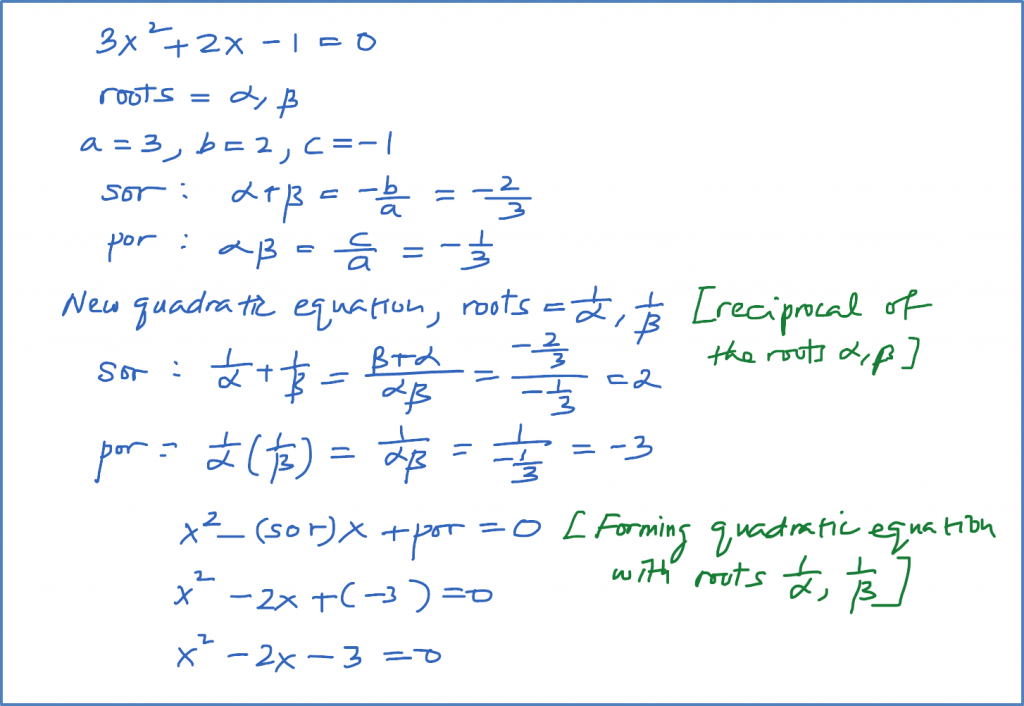# 2.9.2 Quadratic Equation, SPM Practice (Paper 1)

Question 6:
Write and simplify the equation whose roots are the reciprocals of the roots of $3{x}^{2}+2x-1=0$ , without solving the given equation.

Solution:Question 7:
Find the value of p if one root of ${x}^{2}+px+8=0$ is the square of the other.

Solution:Question 8:
If one root of $2{x}^{2}+px+9=0$ is twice the other, find the values of p.

Solution:Question 9:
The roots of the equation are the roots of the equation $2{x}^{2}-x+k=0$ . Find the value of h and k.

Solution:

Question 10:
Find the range of values of p for which the equation $2{x}^{2}+5x+3-p=0$ has two real distinct roots.

Solution:### 1 thought on “2.9.2 Quadratic Equation, SPM Practice (Paper 1)”

1.Question 10:
At b^2 – 4ac > 0, should be 8p, instead of 4p.